# 图像去雾: Single Image Haze Removal Using Dark Channel Prior

## 论文思想

$$I(x) = J(x)t(x) + A(1-t(x)), (1)$$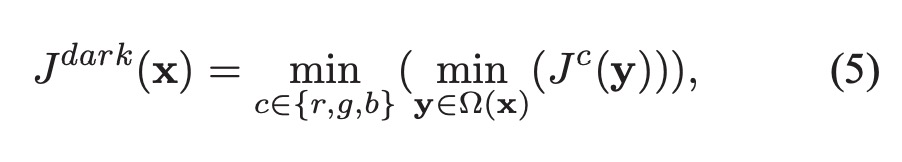Ω(x) 表示以 x 为中心的区域. 区域大小可指定, 求此区域的暗通道相当于在整个RGB通道对该区域进行最小值滤波. 作者通多大量观测得到结论: 如果J 是室外无雾图像, 除开天空区域 $J ^{dark}$ 应该是很小的值，趋近于0.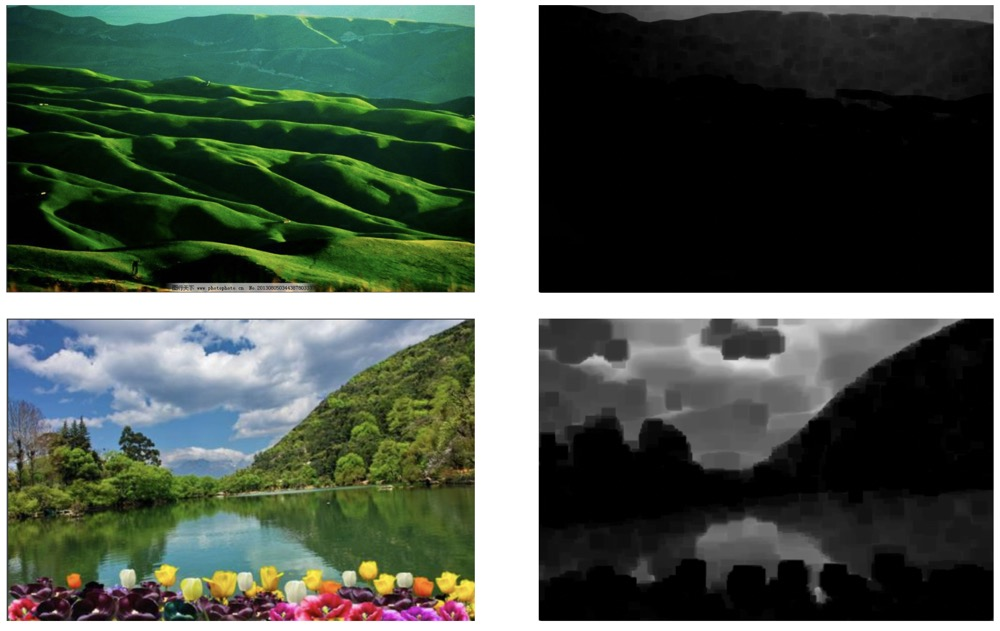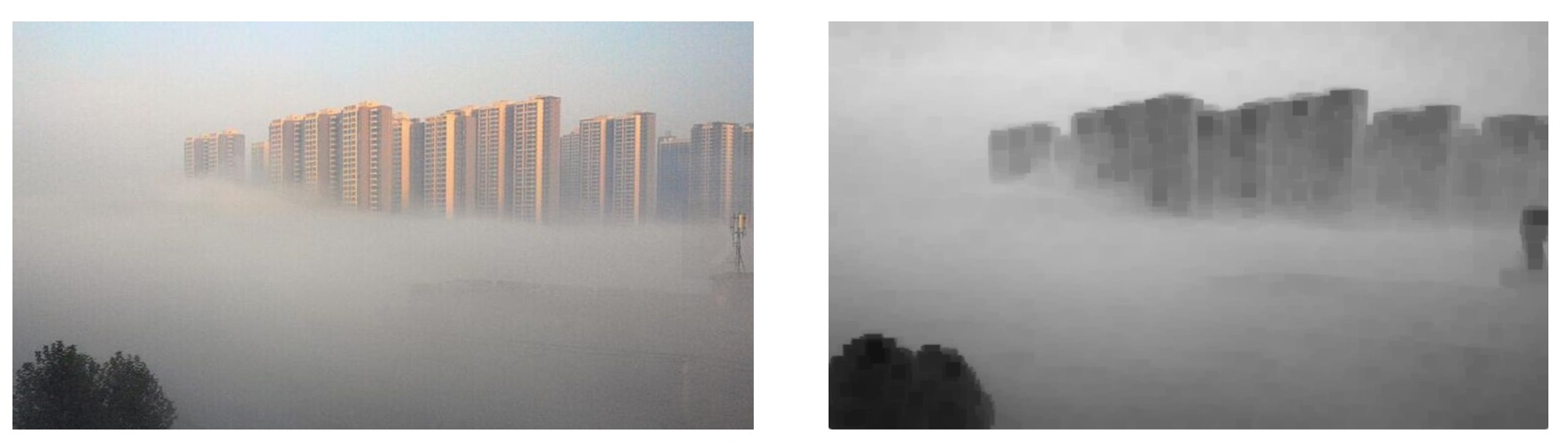## 透射率估计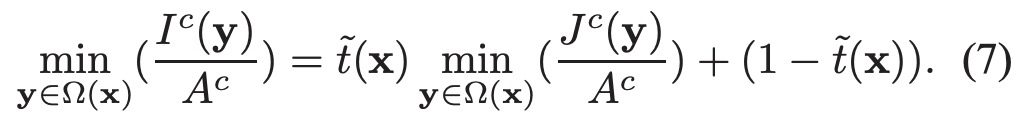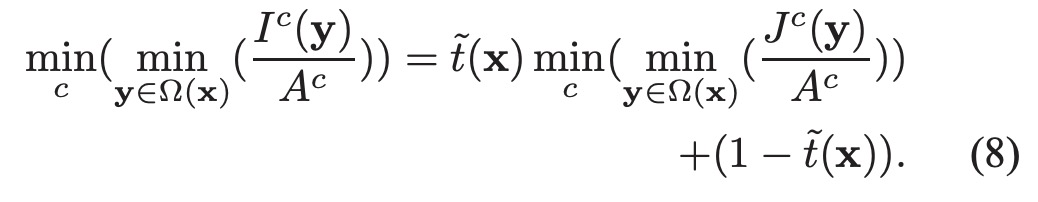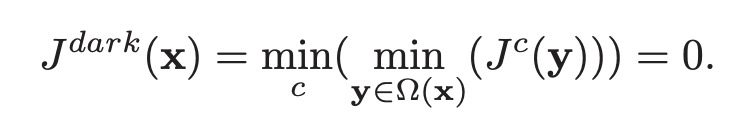$A ^c$ 恒为正数, 因此: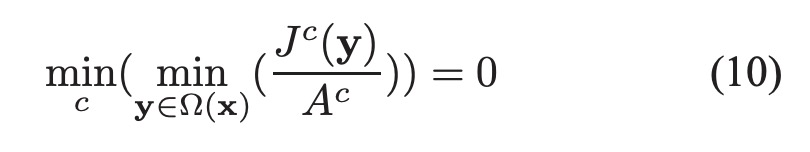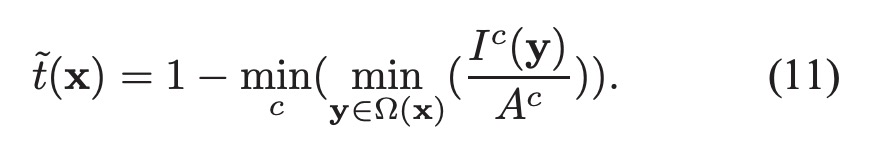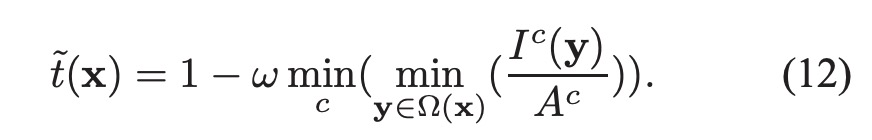## 估计全球大气光

• 从暗通道图中按照亮度的大小取前0.1%的像素。
• 在这些位置中，在原始有雾图像I中寻找对应的具有最高亮度的点的值，作为A值。

## 恢复图像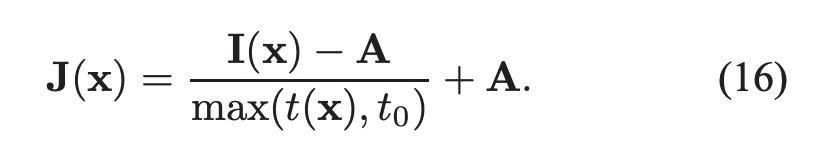## 示例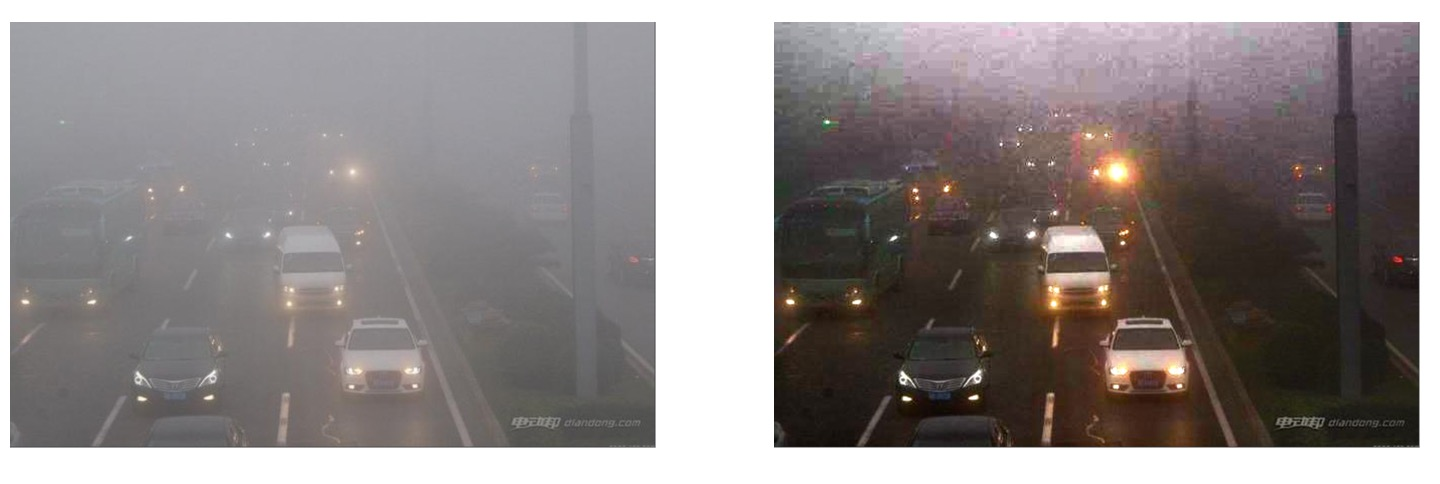## code

import cv2
import math
import numpy as np

def dark_channel(im, sz):
# 暗通道计算
b, g, r = cv2.split(im)
# 取每个像素位置RGB三通道中的最小值
dc = cv2.min(cv2.min(r, g), b)
kernel = cv2.getStructuringElement(cv2.MORPH_RECT, (sz, sz))
# 进行最小值滤波
dark = cv2.erode(dc, kernel)
return dark

def atm_light(im, dark):
# 计算全球大气光的值
[h, w] = im.shape[:2]
imsz = h * w
numpx = int(max(math.floor(imsz / 1000), 1))
darkvec = dark.reshape(imsz, 1)
imvec = im.reshape(imsz, 3)

indices = darkvec.argsort()
indices = indices[imsz - numpx::]

atmsum = np.zeros([1, 3])
# 使用前0.1%的均值, 而不是最大值
for ind in range(1, numpx):
atmsum = atmsum + imvec[indices[ind]]

A = atmsum / numpx
return A

def transmission_estimate(im, A, sz):
# 按照公式（12）计算
omega = 0.95
im3 = np.empty(im.shape, im.dtype)
for ind in range(0, 3):
im3[:, :, ind] = im[:, :, ind] / A[0, ind]

transmission = 1 - omega * dark_channel(im3, sz)
return transmission

def guided_filter(im, p, r, eps):
# http://kaiminghe.com/eccv10/index.html
mean_I = cv2.boxFilter(im, cv2.CV_64F, (r, r))
mean_p = cv2.boxFilter(p, cv2.CV_64F, (r, r))
mean_Ip = cv2.boxFilter(im * p, cv2.CV_64F, (r, r))
cov_Ip = mean_Ip - mean_I * mean_p

mean_II = cv2.boxFilter(im * im, cv2.CV_64F, (r, r))
var_I = mean_II - mean_I * mean_I

a = cov_Ip / (var_I + eps)
b = mean_p - a * mean_I

mean_a = cv2.boxFilter(a, cv2.CV_64F, (r, r))
mean_b = cv2.boxFilter(b, cv2.CV_64F, (r, r))

q = mean_a * im + mean_b
return q

def transmission_refine(im, et):
gray = cv2.cvtColor(im, cv2.COLOR_BGR2GRAY)
gray = np.float64(gray) / 255
r = 60
eps = 0.0001
t = guided_filter(gray, et, r, eps)
return t

def recover(im, t, A, tx=0.1):
# 公式(16)
res = np.empty(im.shape, im.dtype)
t = cv2.max(t, tx)
for ind in range(0, 3):
res[:, :, ind] = (im[:, :, ind] - A[0, ind]) / t + A[0, ind]
return res

if __name__ == '__main__':

I = src.astype('float64') / 255
dark = dark_channel(I, 15)
A = atm_light(I, dark)
te = transmission_estimate(I, A, 15)
t = transmission_refine(src, te)
J = recover(I, t, A, 0.1)

cv2.imshow("dark", dark)
cv2.imshow("t", t)
cv2.imshow('I', src)
cv2.imshow('J', J)
cv2.imwrite("J.png", J * 255)
cv2.waitKey()


## ref

paper

cnblogs图像去雾算法的原理、实现、效果